# Tangents Without Calculus

I always like solving advanced problems with basic methods. For example, many problems that we usually think of as “algebra problems” can be solved by creative thinking without algebra; and some “calculus problems” can be solved using only algebra or geometry. Using simple tools for a big job requires more thought than using “the right tool”, but that’s not a bad thing. I want to look at several ways to find tangents to a parabola without using the derivative, the calculus tool that normally handles this task.

## Slope of the tangent: Algebra

First, a 1996 question using algebra:

Tangent to Parabola

Could you help me me figure out the slopes of two lines tangent to the parabola y = x^2 which pass through the point (2,1)?  The line which passes through (2,1) and (0,0) with a slope of 1/2 seemed like a good bet, but I can't figure out a second tangent.  Thanks - this is for a 10th grade math class.

Here is what Dave has described: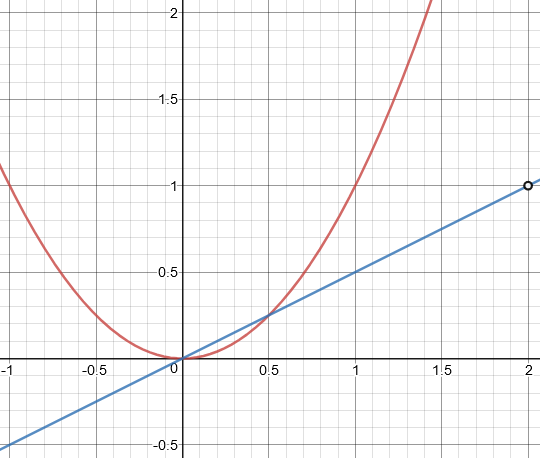(His line may have looked like a tangent at a different scale,but it clearly isn’t, as it passes through the parabola, crossing it twice.)

For a calculus class, this would be easy (sort of); and maybe in some countries that would be covered in 10th grade. But we can use mere algebra. Doctor Jerry took this:

I'm going to assume that solving quadratic equations is within bounds. (There are methods based on calculus, but since you said 10th grade, I'll assume that these are out of bounds for a while.)

All non-vertical lines through (2,1) have the form y - 1 = m(x - 2).  We're looking for values of the slope m for which the line will be tangent to the parabola.  This means that the line will intersect the parabola exactly once.  Thus, when we solve the system

y - 1 = m(x - 2)
y = x^2

we want just one solution.

This is the key to the algebraic method of finding a tangent. We need to find a value of m such that the line will only intersect the parabola once. (If you think about that a bit, you may realize that a vertical line, though not a tangent, would also cross the parabola once. We’ll have to check that idea when we’re finished.)

Substituting from the second equation into the first gives

x^2 - 1 = m(x - 2).

Rearranging this gives

x^2 - mx + (2m - 1) = 0.

This is a quadratic equation, which might have 0, 1, or 2 solutions in x.

This quadratic will have exactly one real root if its discriminant b^2 -4ac is zero.  Thus,

m^2 - 4*1*(2m - 1) = 0.

The roots of this quadratic are 4 + 2*sqrt(3) and 4 - 2*sqrt(3).

These are the slopes of the lines through (2,1) that can be tangent to the given parabola.

Notice that at first we were talking about a quadratic equation in x, where m was a parameter; now we have a quadratic equation in m to solve.

Let’s do that work, to make sure he’s right. The equation simplifies to $$m^2 – 8m + 4 = 0.$$ By the quadratic formula, the solutions are $$m = \frac{8 \pm\sqrt{(-8)^2 – 4(1)(4)}}{2} = \frac{8 \pm\sqrt{48}}{2} = 4 \pm 2\sqrt{3}.$$ Using those slopes for our lines, here are the tangents: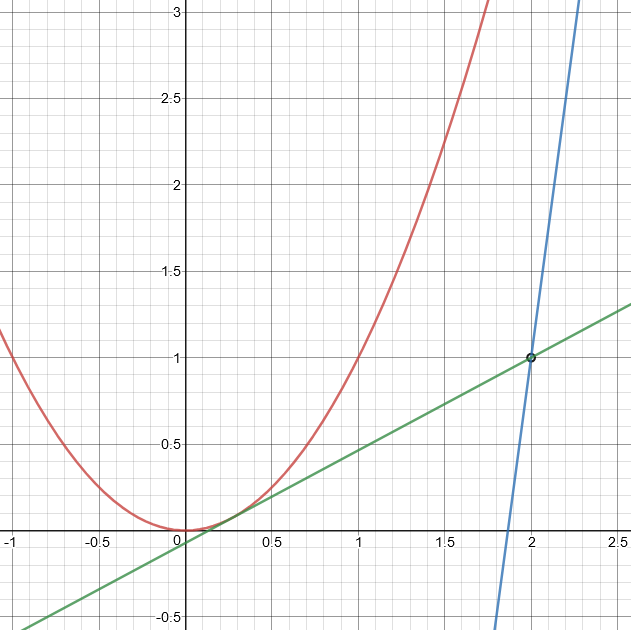Clearly the green line does what Dave’s line didn’t quite do. If we zoomed out, we’d see that the blue line is also tangent.

How about that vertical line I mentioned? Our work has shown that any line even just slightly off vertical will in fact cross the parabola twice, surprising as that may seem; but it doesn’t deal with a vertical line, for which m would have been infinite (that is, really, undefined). That’s why our work didn’t find that line, which is not tangent to the parabola and might have led to an error.

Let’s take this idea a little further. Suppose we want to find the slope of the tangent line to the parabola $$y = x^2$$ at any point $$\left(a, a^2\right)$$. The line with slope m through this point is $$y – a^2 = m(x – a)$$; intersecting this with the parabola by substituting, we have $$x^2 – a^2 = m(x – a)$$. This simplifies to $$x^2 – mx + \left(ma – a^2\right) = 0$$. In order for this to intersect only once, we need the discriminant to be $$m^2 – 4\left(ma – a^2\right) = 0$$. This in turn simplifies to $$m^2 – 4ma + 4a^2 = 0$$, which is $$(m – 2a)^2 = 0$$, so that the solution is $$m = 2a$$. If you know a little calculus, you know that this is, in fact, the derivative of $$y = x^2$$ at $$x = a$$. And we did this with nothing resembling calculus.

## Slope of the tangent: Circles

Now we can look at a 1998 question about a more advanced method, using analytical geometry:

Descartes' Method for Tangents

I've been pretty puzzled by this one. It is really confusing mostly because I have never done a problem like this before because I've only taken Pre-calc. This is my Freshman year in college and this is a Calc I problem. When you do it could you please explain every step and why you did it please?

I will write out the problem for you. Luckily you can figure out what the picture looks like if you read carefully.

Rene Descartes (1596-1650) had the following solution to the construction of tangent lines:

When given the equation of a curve, say the parabola y^2 = 2x, to construct the tangent line to the curve at the point (2,2), we will look at the family of all circles whose center (a,0) is on the x-axis and which passes through the point (2,2).

Only one of these circles will intersect the parabola at only one point (i.e. be tangent to the parabola). When we find this circle, Descartes surmised, then the tangent to that circle will be the tangent to the parabola at (2,2).

Using this method, find the equation of the tangent line.

Here is a picture, showing the parabola in red, point $$A(2,2)$$, and two possible circles, one (with center at $$B$$, in green) that intersects the parabola at two points in the first quadrant (actually a total of four points), and another (with center at $$C$$, in blue) that intersects the parabola at one point in the first quadrant (actually two points total).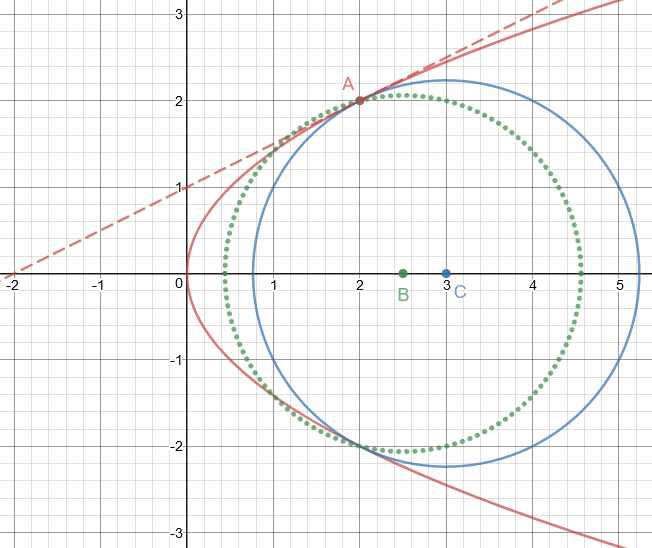The equation of a circle whose center is at (a,0) is:

(x-a)^2 + y^2 = r^2

If the circle passes through (2,2), then you know that:

(2-a)^2 + 2^2 = r^2
r^2 = a^2 - 4*a + 8

Thus the family of all these circles will have equations:

(x-a)^2 + y^2 = a^2 - 4*a + 8

My circles B and C are two members of this family, each one determined by a different value of a.

The parabola has equation y^2 = 2*x. The points of intersection of the parabola and the circle will satisfy both equations, so solve them simultaneously. The fastest way is to substitute y^2 = 2*x in the equation for the circle:

(x-a)^2 + 2*x = a^2 - 4*a + 8
x^2 + (2-2*a)*x + (4*a-8) = 0

Since x = 2 is a solution to this equation, no matter the value of a, x - 2 must be a factor of the lefthand side. Sure enough, this last equation is the same as:

(x - 2)*(x - 2*a + 4) = 0

so x = 2*a - 4 must be the x-coordinate of the other point of intersection. For these two points to coincide, you must have 2 = 2*a - 4, so a = 3.

So here we factored the LHS (which otherwise would have been forbidding) by using the fact that 2 must be a solution, and therefore $$x-2$$ must be a factor, and dividing by that factor using polynomial division. (If you doubt it, try multiplying the factors and verify that you get the right polynomial.) But if there is only one solution (that is, one value of x — which will correspond to two points with positive and negative values of y), the two factors have to be the same, so we get our answer. This point C is, as I showed in the graph, $$(3, 0)$$.

If we hadn’t seen the factoring trick, we could have used the discriminant as in the last problem:

Another way to find a is to realize that for the quadratic in x above to have two equal roots,

0 = B^2 - 4*A*C
= (2-2*a)^2 - 4*(4*a-8)
= 4*(a-3)^2, so
a = 3.

Now we have a circle that is tangent to the parabola. We haven’t yet found the slope of the tangent line. To do that without calculus, we can use the fact that any tangent to a circle is perpendicular to the radius. The radius $$\overline{CA}$$ has slope -2; so the slope of our tangent line is the negative reciprocal, 1/2.

## Reflection property of the parabola: Geometry

Before there was algebra, there was geometry. The parabola was originally defined geometrically. The following question starts with one of several geometric definitions, and looks not just for the tangent line, but for an important property of it:

Parabola: Proving Parallel Rays

Given only the geometric definition of a parabola (all points equally distant from focus and directrix), provide a simple geometric proof that can be explained to sixth graders that all rays emanating from the focus reflect from the parabola parallel to the axis of symmetry.

I'm having difficulty proving to a sixth grader that the tangent line is an angle bisector of the isosceles triangle formed by the focus, the point on the parabola, and the intersection of the perpendicular with the directrix. This proof is necessary to continue the proof that the angle of incidence does indeed equal the angle of reflection.

I'm not sure how much sixth graders can follow, but I think they will be able to see what's going on well enough to satisfy them. Let's take it in two steps. First, see this diagram:Here I have constructed (in green) part of a parabola with the given focus and directrix; for point P I have shown the segments PF to the focus and PQ to the directrix, both with the same length a. In the second step I will be showing that line PM, joining P and the midpoint M of FQ, is tangent to the parabola; for now, it's enough that it looks right. We have an incoming light ray parallel to the axis and perpendicular to the directrix, so it is collinear with PQ. It strikes the parabola at 'angle 1', which is congruent to angle QPM. But angle QPM is congruent to angle FPM, 'angle 2', since PM is the median of isosceles triangle FPQ and consequently also the angle bisector. Therefore 'angle 2' is the direction in which the light will exit, passing through the focus.

I believe you have done this much yourself.

So, if my line PM is the tangent, the reflection property will be true.

Now let's prove that PM is in fact the tangent: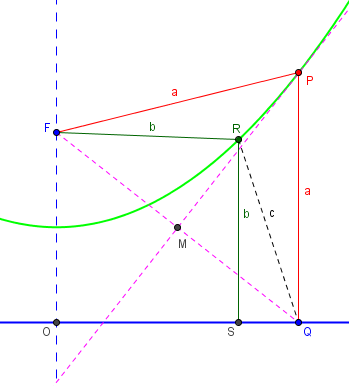Here I again have F, P, and Q, and have drawn PM, which I claim is the tangent. I define the tangent as a line that meets the parabola at P, and nowhere else. That is, I claim that any other point R on the parabola (which is at distance b from the focus and the directrix) is on the same side of PM as the focus.

Look at segment QR, whose length c must be greater than b because it is the hypotenuse of triangle QRS. Now look at triangle QRF. Since c>b, R must be closer to F than to Q, and consequently is on the same side of perpendicular bisector PM as F.

If you put R farther out on the parabola, the same will still be true. Thus all points on the parabola, other than P itself, are on the same side of PM, and the latter is the tangent we are looking for.

Here is the picture when R is farther out: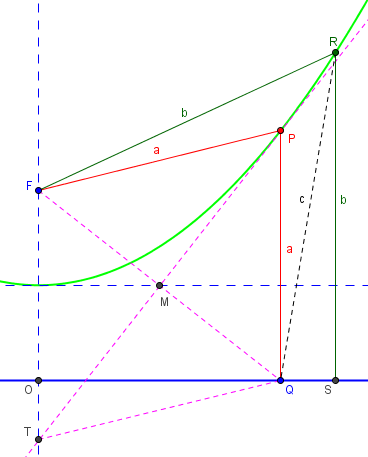In a geometry class I would have invoked a few specific theorems to make my conclusions here, but I  tried to express everything in fairly obvious terms.

## Slope of the tangent: Geometry

Let’s look at one more thing in this diagram: What is the slope of the tangent line?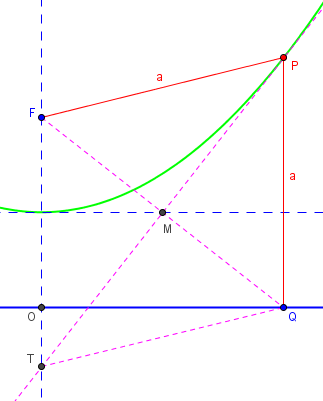I’ve added in the horizontal line through M, which is midway between the focus F and the directrix OQ; it passes through the vertex of the parabola (making it the x-axis). It is easy to see that if P has coordinates $$\left(x, x^2\right)$$, then M has coordinates ($$\left(\frac{x}{2}, 0\right)$$. The slope is therefore $$\displaystyle \frac{x^2}{\frac{x}{2}} = 2x$$, just as we know from calculus. We can also see that if you ever want to draw a tangent to a parabola at a given point, you just have to make it pass through the point on the x-axis halfway to the given point.

For an alternative demonstration of the reflection property, using calculus and trigonometry, see

Reflections in Parabolic Mirrors

### 2 thoughts on “Tangents Without Calculus”

1.Great help

This site uses Akismet to reduce spam. Learn how your comment data is processed.